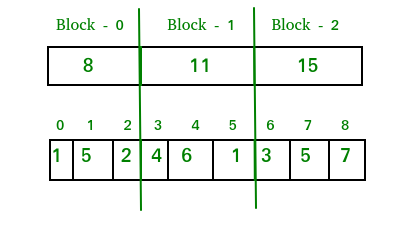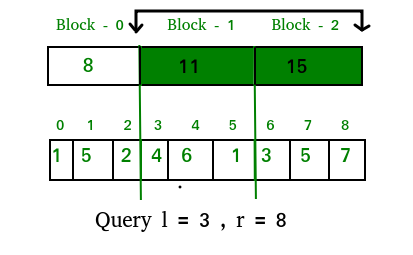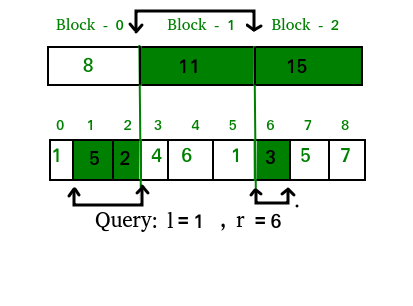# Sqrt (or Square Root) Decomposition Technique | Set 1 (Introduction)

• Difficulty Level : Medium
• Last Updated : 21 Aug, 2022

Sqrt (or Square Root) Decomposition Technique is one of the most common query optimization technique used by competitive programmers. This technique helps us to reduce Time Complexity by a factor of sqrt(n)
The key concept of this technique is to decompose given array into small chunks specifically of size sqrt(n).
Let’s say we have an array of n elements and we decompose this array into small chunks of size sqrt(n). We will be having exactly sqrt(n) such chunks provided that n is a perfect square. Therefore, now our array on n elements is decomposed into sqrt(n) blocks, where each block contains sqrt(n) elements (assuming size of array is perfect square).
Let’s consider these chunks or blocks as an individual array each of which contains sqrt(n) elements and you have computed your desired answer(according to your problem) individually for all the chunks. Now, you need to answer certain queries asking you the answer for the elements in range l to r(l and r are starting and ending indices of the array) in the original n sized array.
The naive approach is simply to iterate over each element in range l to r and calculate its corresponding answer. Therefore, the Time Complexity per query will be O(n).
Sqrt Decomposition Trick : As we have already precomputed the answer for all individual chunks and now we need to answer the queries in range l to r. Now we can simply combine the answers of the chunks that lie in between the range l to r in the original array. So, if we see carefully here we are jumping sqrt(n) steps at a time instead of jumping 1 step at a time as done in naive approach. Let’s just analyze its Time Complexity and implementation considering the below problem :-

```Problem :
Given an array of n elements. We need to answer q
queries telling the sum of elements in range l to
r in the array. Also the array is not static i.e
the values are changed via some point update query.

Range Sum Queries are of form : Q l r ,
where l is the starting index r is the ending
index

Point update Query is of form : U idx val,
where idx is the index to update val is the
updated value```

Let us consider that we have an array of 9 elements.
A[] = {1, 5, 2, 4, 6, 1, 3, 5, 7}
Let’s decompose this array into sqrt(9) blocks, where each block will contain the sum of elements lying in it. Therefore now our decomposed array would look like this :Till now we have constructed the decomposed array of sqrt(9) blocks and now we need to print the sum of elements in a given range. So first let’s see two basic types of overlap that a range query can have on our array :-

Range Query of type 1 (Given Range is on Block Boundaries) :In this type the query, the range may totally cover the continuous sqrt blocks. So we can easily answer the sum of values in this range as the sum of completely overlapped blocks.
So answer for above query in the described image will be : ans = 11 + 15 = 26
Time Complexity: In the worst case our range can be 0 to n-1(where n is the size of array and assuming n to be a perfect square). In this case all the blocks are completely overlapped by our query range. Therefore,to answer this query we need to iterate over all the decomposed blocks for the array and we know that the number of blocks = sqrt(n). Hence, the complexity for this type of query will be O(sqrt(n)) in worst case.

Range Query of type 2 (Given Range is NOT on boundaries)We can deal these type of queries by summing the data from the completely overlapped decomposed blocks lying in the query range and then summing elements one by one from the original array whose corresponding block is not completely overlapped by the query range.
So answer for above query in the described image will be : ans = 5 + 2 + 11 + 3 = 21
Time Complexity: Let’s consider a query [l = 1 and r = n-2] (n is the size of the array and has a 0 based indexing). Therefore, for this query exactly ( sqrt(n) – 2 ) blocks will be completely overlapped where as the first and last block will be partially overlapped with just one element left outside the overlapping range. So, the completely overlapped blocks can be summed up in ( sqrt(n) – 2 ) ~ sqrt(n) iterations, whereas first and last block are needed to be traversed one by one separately. But as we know that the number of elements in each block is at max sqrt(n), to sum up last block individually we need to make,
(sqrt(n)-1) ~ sqrt(n) iterations and same for the last block.
So, the overall Complexity = O(sqrt(n)) + O(sqrt(n)) + O(sqrt(n)) = O(3*sqrt(N)) = O(sqrt(n))

Update Queries(Point update) :

In this query we simply find the block in which the given index lies, then subtract its previous value and add the new updated value as per the point update query.
Time Complexity : O(1)

Implementation :

The implementation of the above trick is given below

## C++

 `// C++ program to demonstrate working of Square Root` `// Decomposition.` `#include "iostream"` `#include "math.h"` `using` `namespace` `std;`   `#define MAXN 10000` `#define SQRSIZE  100`   `int` `arr[MAXN];               ``// original array` `int` `block[SQRSIZE];          ``// decomposed array` `int` `blk_sz;                      ``// block size`   `// Time Complexity : O(1)` `void` `update(``int` `idx, ``int` `val)` `{` `    ``int` `blockNumber = idx / blk_sz;` `    ``block[blockNumber] += val - arr[idx];` `    ``arr[idx] = val;` `}`   `// Time Complexity : O(sqrt(n))` `int` `query(``int` `l, ``int` `r)` `{` `    ``int` `sum = 0;` `    ``while` `(l

## Java

 `// Java program to demonstrate working of ` `// Square Root Decomposition.` `import` `java.util.*;`   `class` `GFG ` `{`   `static` `int` `MAXN = ``10000``;` `static` `int` `SQRSIZE = ``100``;`   `static` `int` `[]arr = ``new` `int``[MAXN];             ``// original array` `static` `int` `[]block = ``new` `int``[SQRSIZE];         ``// decomposed array` `static` `int` `blk_sz;                             ``// block size`   `// Time Complexity : O(1)` `static` `void` `update(``int` `idx, ``int` `val)` `{` `    ``int` `blockNumber = idx / blk_sz;` `    ``block[blockNumber] += val - arr[idx];` `    ``arr[idx] = val;` `}`   `// Time Complexity : O(sqrt(n))` `static` `int` `query(``int` `l, ``int` `r)` `{` `    ``int` `sum = ``0``;` `    ``while` `(l < r && l % blk_sz != ``0` `&& l != ``0``)` `    ``{` `        ``// traversing first block in range` `        ``sum += arr[l];` `        ``l++;` `    ``}` `    ``while` `(l+blk_sz-``1` `<= r)` `    ``{` `        ``// traversing completely` `        ``// overlapped blocks in range` `        ``sum += block[l / blk_sz];` `        ``l += blk_sz;` `    ``}` `    ``while` `(l <= r)` `    ``{` `        ``// traversing last block in range` `        ``sum += arr[l];` `        ``l++;` `    ``}` `    ``return` `sum;` `}`   `// Fills values in input[]` `static` `void` `preprocess(``int` `input[], ``int` `n)` `{` `    ``// initiating block pointer` `    ``int` `blk_idx = -``1``;`   `    ``// calculating size of block` `    ``blk_sz = (``int``) Math.sqrt(n);`   `    ``// building the decomposed array` `    ``for` `(``int` `i = ``0``; i < n; i++)` `    ``{` `        ``arr[i] = input[i];` `        ``if` `(i % blk_sz == ``0``)` `        ``{` `            ``// entering next block` `            ``// incrementing block pointer` `            ``blk_idx++;` `        ``}` `        ``block[blk_idx] += arr[i];` `    ``}` `}`   `// Driver code` `public` `static` `void` `main(String[] args) ` `{` `    `  `    ``// We have used separate array for input because` `    ``// the purpose of this code is to explain SQRT` `    ``// decomposition in competitive programming where` `    ``// we have multiple inputs.` `    ``int` `input[] = {``1``, ``5``, ``2``, ``4``, ``6``, ``1``, ``3``, ``5``, ``7``, ``10``};` `    ``int` `n = input.length;`   `    ``preprocess(input, n);`   `    ``System.out.println(``"query(3, 8) : "` `+` `                        ``query(``3``, ``8``));` `    ``System.out.println(``"query(1, 6) : "` `+` `                        ``query(``1``, ``6``));` `    ``update(``8``, ``0``);` `    ``System.out.println(``"query(8, 8) : "` `+ ` `                        ``query(``8``, ``8``));` `}` `} `   `// This code is contributed by PrinciRaj1992`

## Python 3

 `# Python 3 program to demonstrate working of Square Root` `# Decomposition.` `from` `math ``import` `sqrt`   `MAXN ``=` `10000` `SQRSIZE ``=` `100`   `arr ``=` `[``0``]``*``(MAXN)         ``# original array` `block ``=` `[``0``]``*``(SQRSIZE)     ``# decomposed array` `blk_sz ``=` `0`                 `# block size`   `# Time Complexity : O(1)` `def` `update(idx, val):` `    ``blockNumber ``=` `idx ``/``/` `blk_sz` `    ``block[blockNumber] ``+``=` `val ``-` `arr[idx]` `    ``arr[idx] ``=` `val`   `# Time Complexity : O(sqrt(n))` `def` `query(l, r):` `    ``sum` `=` `0` `    ``while` `(l < r ``and` `l ``%` `blk_sz !``=` `0` `and` `l !``=` `0``):` `        `  `        ``# traversing first block in range` `        ``sum` `+``=` `arr[l]` `        ``l ``+``=` `1` `    `  `    ``while` `(l ``+` `blk_sz ``-` `1` `<``=` `r):` `        `  `        ``# traversing completely overlapped blocks in range` `        ``sum` `+``=` `block[l``/``/``blk_sz]` `        ``l ``+``=` `blk_sz` `    `  `    ``while` `(l <``=` `r):` `        `  `        ``# traversing last block in range` `        ``sum` `+``=` `arr[l]` `        ``l ``+``=` `1` `    `  `    ``return` `sum` `    `  `# Fills values in input[]` `def` `preprocess(``input``, n):` `    `  `    ``# initiating block pointer` `    ``blk_idx ``=` `-``1`   `    ``# calculating size of block` `    ``global` `blk_sz` `    ``blk_sz ``=` `int``(sqrt(n))`   `    ``# building the decomposed array` `    ``for` `i ``in` `range``(n):` `        ``arr[i] ``=` `input``[i];` `        ``if` `(i ``%` `blk_sz ``=``=` `0``):` `            `  `            ``# entering next block` `            ``# incrementing block pointer` `            ``blk_idx ``+``=` `1``;` `        `  `        ``block[blk_idx] ``+``=` `arr[i]`   `# Driver code`   `# We have used separate array for input because` `# the purpose of this code is to explain SQRT` `# decomposition in competitive programming where` `# we have multiple inputs.` `input``=` `[``1``, ``5``, ``2``, ``4``, ``6``, ``1``, ``3``, ``5``, ``7``, ``10``]` `n ``=` `len``(``input``)`   `preprocess(``input``, n)`   `print``(``"query(3,8) : "``,query(``3``, ``8``))` `print``(``"query(1,6) : "``,query(``1``, ``6``))` `update(``8``, ``0``)` `print``(``"query(8,8) : "``,query(``8``, ``8``))`   `# This code is contributed by Sanjit_Prasad`

## C#

 `// C# program to demonstrate working of ` `// Square Root Decomposition. ` `using` `System;` `    `  `class` `GFG ` `{` `static` `int` `MAXN = 10000;` `static` `int` `SQRSIZE = 100;`   `static` `int` `[]arr = ``new` `int``[MAXN];             ``// original array` `static` `int` `[]block = ``new` `int``[SQRSIZE];         ``// decomposed array` `static` `int` `blk_sz;                             ``// block size`   `// Time Complexity : O(1)` `static` `void` `update(``int` `idx, ``int` `val)` `{` `    ``int` `blockNumber = idx / blk_sz;` `    ``block[blockNumber] += val - arr[idx];` `    ``arr[idx] = val;` `}`   `// Time Complexity : O(sqrt(n))` `static` `int` `query(``int` `l, ``int` `r)` `{` `    ``int` `sum = 0;` `    ``while` `(l < r && l % blk_sz != 0 && l != 0)` `    ``{` `        ``// traversing first block in range` `        ``sum += arr[l];` `        ``l++;` `    ``}` `    ``while` `(l + blk_sz - 1 <= r)` `    ``{` `        ``// traversing completely` `        ``// overlapped blocks in range` `        ``sum += block[l / blk_sz];` `        ``l += blk_sz;` `    ``}` `    ``while` `(l <= r)` `    ``{` `        ``// traversing last block in range` `        ``sum += arr[l];` `        ``l++;` `    ``}` `    ``return` `sum;` `}`   `// Fills values in input[]` `static` `void` `preprocess(``int` `[]input, ``int` `n)` `{` `    ``// initiating block pointer` `    ``int` `blk_idx = -1;`   `    ``// calculating size of block` `    ``blk_sz = (``int``) Math.Sqrt(n);`   `    ``// building the decomposed array` `    ``for` `(``int` `i = 0; i < n; i++)` `    ``{` `        ``arr[i] = input[i];` `        ``if` `(i % blk_sz == 0)` `        ``{` `            ``// entering next block` `            ``// incrementing block pointer` `            ``blk_idx++;` `        ``}` `        ``block[blk_idx] += arr[i];` `    ``}` `}`   `// Driver code` `public` `static` `void` `Main(String[] args) ` `{` `    `  `    ``// We have used separate array for input because` `    ``// the purpose of this code is to explain SQRT` `    ``// decomposition in competitive programming where` `    ``// we have multiple inputs.` `    ``int` `[]input = {1, 5, 2, 4, 6, 1, 3, 5, 7, 10};` `    ``int` `n = input.Length;`   `    ``preprocess(input, n);`   `    ``Console.WriteLine(``"query(3, 8) : "` `+` `                       ``query(3, 8));` `    ``Console.WriteLine(``"query(1, 6) : "` `+` `                       ``query(1, 6));` `    ``update(8, 0);` `    ``Console.WriteLine(``"query(8, 8) : "` `+ ` `                       ``query(8, 8));` `}` `}`   `// This code is contributed by 29AjayKumar`

## Javascript

 ``

Output

```query(3,8) : 26
query(1,6) : 21
query(8,8) : 0```

Time Complexity: O(n)

Auxiliary Space: O(MAXN), since MAXN extra space has been taken.
Note : The above code works even if n is not perfect square. In the case, the last block will contain even less number of elements than sqrt(n), thus reducing the number of iterations.
Let’s say n = 10. In this case we will have 4 blocks first three block of size 3 and last block of size 1.
This article is contributed by Nitish Kumar. If you like GeeksforGeeks and would like to contribute, you can also write an article using write.geeksforgeeks.org or mail your article to review-team@geeksforgeeks.org. See your article appearing on the GeeksforGeeks main page and help other Geeks.
Please write comments if you find anything incorrect, or you want to share more information about the topic discussed above.

My Personal Notes arrow_drop_up
Recommended Articles
Page :